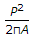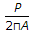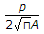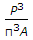# Civil Engineering - Water Resources Engineering

### Exercise :: Water Resources Engineering - Section 2

21.

The best instrument for measuring the velocity of a stream flow is

 A. pitot tube B. Price's current meter C. surface float D. sub-surface float.

Explanation:

No answer description available for this question. Let us discuss.

22.

The average mean velocity of a stream having depth h, may be obtained by taking the average of the readings of a current meter at a depth of

 A. 0.1 h and 0.9 h B. 0.2 h and 0.8 h C. 0.3 h and 0.7 h D. 0.4 h and 0.6 h.

Explanation:

No answer description available for this question. Let us discuss.

23.

If the velocities of flow of a stream of 10 m depth recorded by a current meter at depths of 2 m and 8 m are 0.7 m and 0.3 m respectively, the discharge per unit width of the stream in cubic metres, is

 A. 2 B. 3 C. 4 D. 5 E. 6

Explanation:

No answer description available for this question. Let us discuss.

24.

If P and A are the perimeter and area of a drainage basin, its compactness coefficient, is

 A.B.C.D.Explanation:

No answer description available for this question. Let us discuss.

25.

The time required by rain water to reach the outlet of drainage basin, is generally called

 A. time of concentration B. time of overland flow C. concentration time of overland flow D. duration of the rainfall E. none of these.